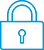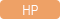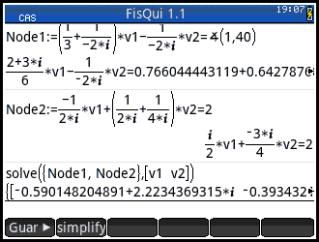cancel
Showing results for
Did you mean:It has been a while since anyone has replied. Simply ask a new question if you would like to start the discussion again.New member
1 0 0 0
Message 1 of 3
642
Flag Post

# Node 1: (1/3+1/-j2)V1-(1/-j2)V2=1angle40deg Node 2: -(1/j2)V1+(1/1j2+1/j4+1/6)V2=2angle0degress. ???HP Recommended
HP Prime Calculator

Node 1: (1/3+1/-j2)V1-(1/-j2)V2=1Aangle40deg Node 2: -(1/j2)V1+(1/1j2+1/j4+1/6)V2=2Aangle0degress.

How do I input (HP Prime calculator) this to solve for V1 and V2?

Tags (1)
2 REPLIES 2Level 6
252 251 42 78
Message 2 of 3
Flag PostHP Recommended

Hello,

I am not sure that I understood your question correctly, so I will make some assumptions. Hopefully, even if they are incorrect, I hope that I will give you enough information to still solve the problem.

Anyhow, here are the assumptions that I made.

1angle40deg means 1 degree 40 minutes, or as expressed as a number 1+40/60

j is the typical electrical engineering way to "name" imaginary number sqrt(-1) usually named "i" by mathematicians.

j2 means (in mathematicians terms) i*2

With these assumtions, here is what I entered to solve the problem:

in CAS mode (press the CAS key): use the csolve function:

csolve([(((1/3)+(1/(-2*i)))*V1-(1/(-2*i))*V2) = (1+(40/60)),((-1/(i*2))*V1+((1/(2*i))+(1/(i*4))+(1/6))*V2) = 2],[V1,V2])

and get the result:

{[(476+6*i)/313,(48+12*i)/(13-12*i)]}

which are the values for V1 and V2

if I am wrong on the assumptions that j2 means i*2, then use the power function and you get the result:

{[41/22,9/11]}

Cyrille

I am an HP EmployeeLevel 4
138 122 6 17
Message 3 of 3
Flag PostHP Recommended

Hello JaspercussionIt's important to pay attention on the following details:

1) 2 angle 0 has been typed as 2, just that. Angle 0 usually bugs.

2) use "i" instead of "j". As you're solving an electric circuit, "i" means current and "j" is used instead. But for means of calculators, you must use "i" for impedance.

3) the angle sign is found in Shift X.

4) "solve" can comprehend as many node equations as you want, but all them must be in a list between {}. The desired variables to solve, such as v1 and v2, must be inside [], as they're coordinated.

Please try it yourself and tell me.

Regards,

Ciro.

† The opinions expressed above are the personal opinions of the authors, not of HP. By using this site, you accept the Terms of Use and Rules of Participation